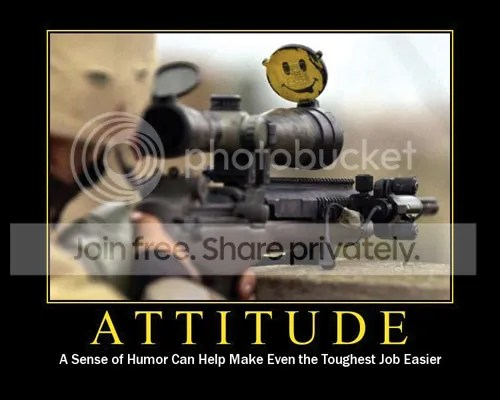1+20+20+9+20+21+4+5 = ATTITUDE

A silly fact that I have just known today on my character development class.
A = 1; B = 2; C = 3; D = 4; E = 5; … ; X = 24; Y = 25; Z = 26.

SKILL = 19+11+9+12+12 = 63
KNOWLEDGE = 11+14+15+23+12+5+4+7+5 = 96
HARD WORK = 8+1+18+4+23+15+18+11 = 98

You might have all the SKILL that no one can not beat, you might be very capable of everything with the KNOWLEDGE you have learned, and you might can work your ass off with HARD WORK to go to the top.

ATTITUDE = 1+20+20+9+20+21+4+5 = 100

But remember one thing, only ATTITUDE that makes you survive the battles. Build up your attitude and do some kick ass stuffs! TRUST ME IT WORKS.[picture taken from photobucket]